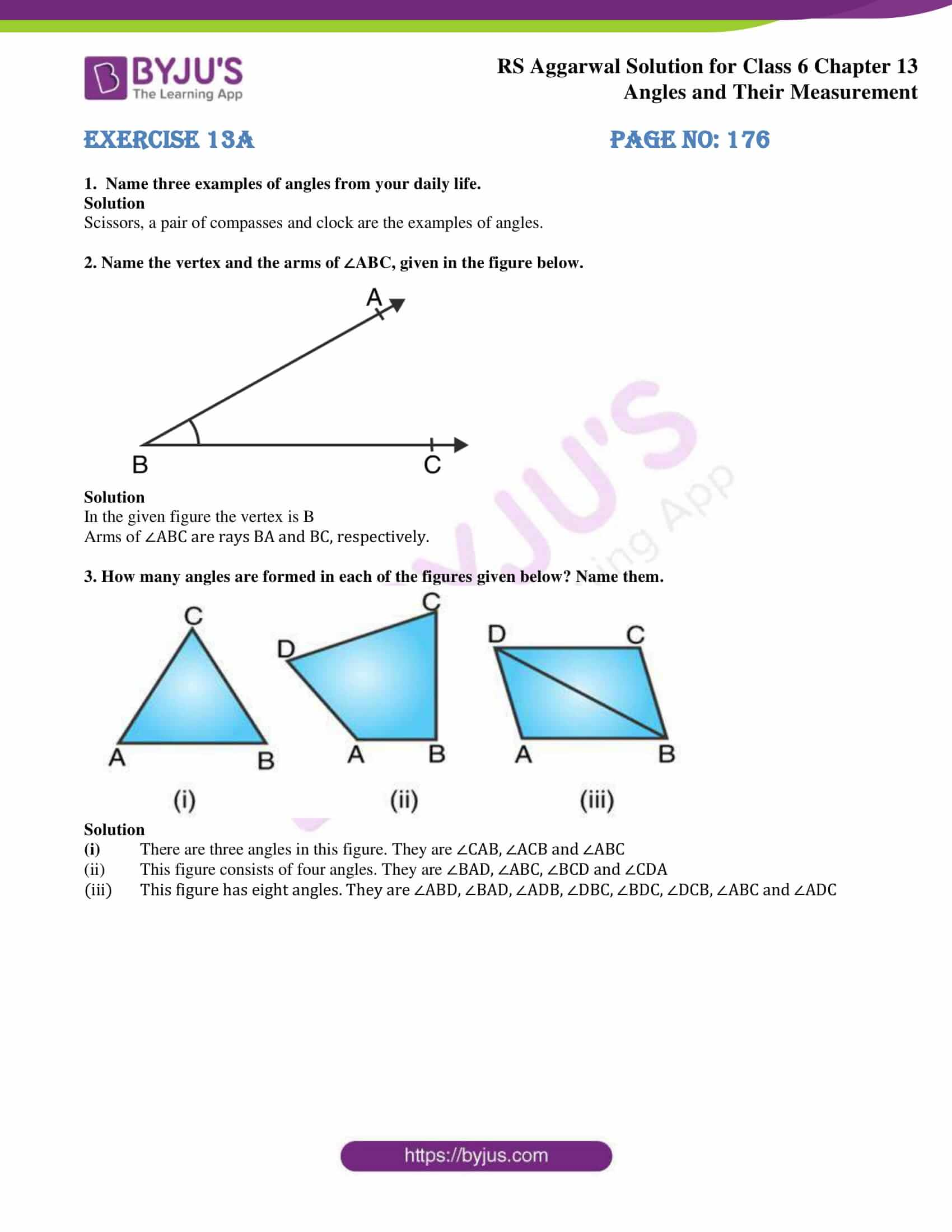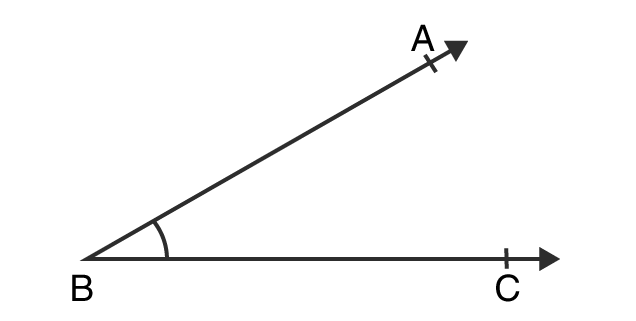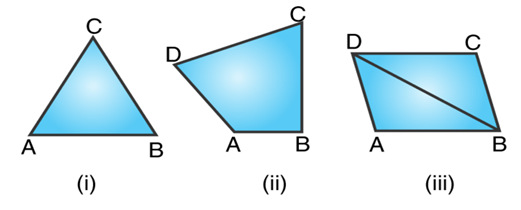# RS Aggarwal Solutions for Class 6 Chapter 13 Angles and their Measurement Exercise 13A

Exercise 13A of chapter 13 provides students with knowledge about the concepts like angles and ways to find them. The figure which is formed by two rays with the same initial point is called an angle. The students can use the PDF of RS Aggarwal Solutions while solving the exercise wise problems to get their doubts cleared. The students can make use of RS Aggarwal Solutions for Class 6 Chapter 13 Angles and their Measurement Exercise 13A PDF to ace the exam.

## Download PDF of RS Aggarwal Solutions for Class 6 Chapter 13 Angles and their Measurement Exercise 13A### Access answers to Maths RS Aggarwal Solutions for Class 6 Chapter 13 Angles and their Measurement Exercise 13A

1. Name three examples of angles from your daily life.

Solution

Scissors, a pair of compasses and clock are the examples of angles.

2. Name the vertex and the arms of ∠ABC, given in the figure below.Solution

In the given figure the vertex is B

Arms of ∠ABC are rays BA and BC, respectively.

3. How many angles are formed in each of the figures given below? Name them.Solution

(i) There are three angles in this figure. They are ∠CAB, ∠ACB and ∠ABC

(ii) This figure consists of four angles. They are ∠BAD, ∠ABC, ∠BCD and ∠CDA

(iii) This figure has eight angles. They are ∠ABD, ∠BAD, ∠ADB, ∠DBC, ∠BDC, ∠DCB, ∠ABC and ∠ADC

### Access other exercises of RS Aggarwal Solutions for Class 6 Chapter 13 Angles and their Measurement

Exercise 13D Solutions